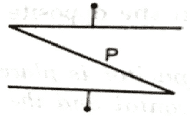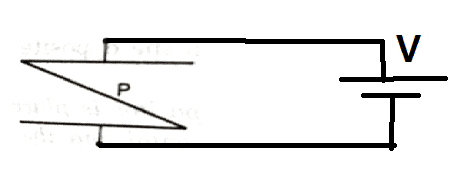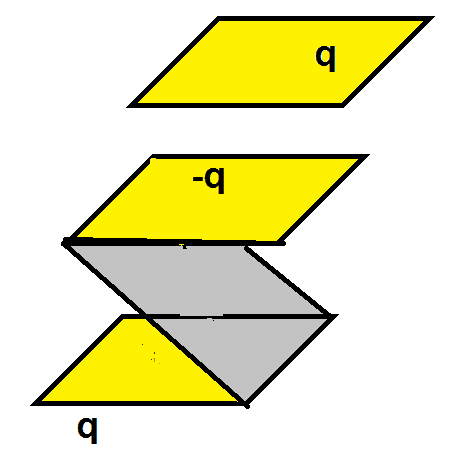# Capacitance of a parallel plate capacitor with a metal plate kept inside

Pushoam
Homework Statement:
A thin metal plate P is inserted between the plates of a parallel plate capacitor of capacitance C in such a way that its edges touch the two plates. The capacitance now becomes (a) 0 (b) infinity.
Relevant Equations:
$$C=\frac Q V$$
Because of the plate P, the capacitor becomes a piece of conductor. It contains zero net charge and has 0 potential difference. Hence, the capacitance is ## \frac 0 0 # # that is undefined.

The capacitance of a capacitor is defined as its capacity to store charge when a potential difference is applied across the plates. Here, the plates cannot store net charge. So the net stored charge is zero. Hence, it's capacitance should be zero.

Last edited:

Homework Helper
Gold Member
Homework Statement:: A thin metal plate P is inserted between the plates of a parallel plate capacitor of capacitance C in such a way that its edges touch the two plates. The capacitance now becomes (a) 0 (b) infinity.
Relevant Equations:: $$C=\frac Q V$$

Because of the plate P, the capacitor becomes a piece of conductor. It contains zero net charge and has 0 potential difference. Hence, the capacitance is ## \frac 0 0 # # that is undefined.

The capacitance of a capacitor is defined as its capacity to store charge when a potential difference is applied across the plates. Here, the plates cannot store net charge. So the net stored charge is zero. Hence, it's capacitance should be zero.
A capacitor is defined as two separate conductors separated in space such that if one transfers charge ##Q## from one conductor to the other, a potential difference ##V## will exist between the two. The capacitance is defined by the equation you quoted and is a geometrical property. It depends on the shape and configuration of the two conductors, not on whether there is charge ##+Q## on one and ##-Q## on the other. An uncharged capacitor still has capacitance.

So your justification that capacitance goes to zero because the modified parallel plate capacitor does not store charge is incorrect. You can always put charge on a conductor in which case there will be a capacitance with the "other conductor" being at infinity. Then the charge you put on this conductor divided by the potential difference between it and infinity is the capacitance.

In view of all this, it appears that neither of the given choices is correct. This does not look like a well crafted problem to me. Is this in a textbook?

•vela and Steve4Physics
Pushoam
Yes, it is in a book.I think here there is no other conductor at infinity.
I am asked to find the capacitance of the parallel plate capacitor when a metallic plate P is inserted.
So, I put a battery across the two terminal and calculate C. $$C=\frac Q V$$Now, Q = 0, but V, too, is 0 ideally and close to 0 practically. So, the capacitance of the parallel plate capacitor now becomes 0.

Gold Member
What an odd question. I'm with you, I think the whole thing is undefined using normal circuit assumptions (mostly that conductors have no impedance). OTOH, I am curious if someone has a good story about why the question does make sense.

PushoamI have got another solution for this.
I put a metal plate with charge q above the capacitor.
Due to induction, the upper plate gets charge -q and the below plate gets charge q while the potential difference between the plates i.e. V is 0.
Hence, ## C = \frac q V = \frac q 0 = \infty ##.

The book gives the answer as ##\infty##.

•DaveE
Pushoam
Thanks.

Homework Helper
Gold Member
2022 Award
View attachment 321946
I have got another solution for this.
I put a metal plate with charge q above the capacitor.
Due to induction, the upper plate gets charge -q and the below plate gets charge q while the potential difference between the plates i.e. V is 0.
Hence, ## C = \frac q V = \frac q 0 = \infty ##.

The book gives the answer as ##\infty##.
By that argument any single conductor (e.g. a metal cube) has an infinite capacitance!

Pushoam
By that argument any single conductor (e.g. a metal cube) has an infinite capacitance!
Yes, it has.

Homework Helper
Gold Member
I think here there is no other conductor at infinity.
There may be no other conductor at infinity but at infinity, all electric fields are zero and infinity is an equipotential. So if you put some charge ##+Q## on a conductor of any shape you will raise its electric potential relative to infinity. This means a potential difference ##V## between infinity and the conductor. The ratio ##Q/V## is the capacitance of the conductor.

•Pushoam
Homework Helper
Gold Member
2022 Award
By that argument any single conductor (e.g. a metal cube) has an infinite capacitance!
Yes, it has.
The capacitance of a simple 2-plate capacitor is (using the usual symbols) ##C = \frac {\epsilon_r\epsilon_0 A}d## where ##\epsilon_r## is the relative permittivity (dielectric constant) of the material between the plates. For a perfect conductor, ##\epsilon_r=∞## which is, essentially, why the book answer is ##C=∞##.

But the underlying problem is that a '2-plate' capacitor (whatever the shape of the 'plates') must have a dielectric (insulator) between the plates. If you internally 'short-circuit' the 2 plates, as in the question, you no longer have a 2-plate capacitor - you have a single conducting object.

By convention, when considering the capacitance of a single conducting object, the relevant potential difference is between the conductor and ‘infinity’, as already noted by @kuruman in Post #2. With this convention, for example the accepted capacitance of an isolated conducting sphere of radius ##r## is ##C= 4\pi \epsilon_0 r##.

•Pushoam and nasu
Homework Helper
Gold Member
View attachment 321946
I have got another solution for this.
I put a metal plate with charge q above the capacitor.
Due to induction, the upper plate gets charge -q and the below plate gets charge q while the potential difference between the plates i.e. V is 0.
Hence, ## C = \frac q V = \frac q 0 = \infty ##.

The book gives the answer as ##\infty##.
You seem to labor under the impression that without stored charge and potential difference a capacitor does not have capacitance. That is not the case. As stated earlier, capacitance is a geometric property that does not depend on whether there is a charge on the capacitor or a potential difference between the conductors forming the capacitor. Capacitance is like mass or moment of inertia. An object does not need to accelerate in order to have mass or rotate in order to have moment of inertia.

For example, the capacitance of a parallel plate capacitor is (approximately) ##C=\frac{\epsilon_0 A}{d}## and there is no ##Q## or ##V## in the expression. However, to get that expression one has to perform a thought experiment that can be found in any introductory textbook.
1. Charge ##Q## is removed from one conductor forming the capacitor and placed on the other.
2. The potential difference ##V## is measured or calculated between the two conductors.
3. The capacitance is the ratio ##Q/V##.
This cannot happen with the "Z"-shaped conductor that you have. If you start with no charge on it and move ##+Q## from the top of the "Z" to the bottom, you will create a potential difference between top and bottom and the charge will go back where it came from. However, the "Z"-shaped piece and the other plate above it do form a capacitor and you can find its capacitance using the procedure outlined above. Or you can consider the "Z"-shaped alone, bring some charge from infinity and put it on then find the capacitance relative to infinity as mentioned earlier.

•Pushoam
Pushoam
The capacitance of a simple 2-plate capacitor is (using the usual symbols) ##C = \frac {\epsilon_r\epsilon_0 A}d## where ##\epsilon_r## is the relative permittivity (dielectric constant) of the material between the plates. For a perfect conductor, ##\epsilon_r=∞## which is, essentially, why the book answer is ##C=∞##.
I understood that this is what my book meant and after the insertion of the metal plate P, the parallel plate capacitor becomes a "z" shape piece of conductor and its capacitance has to be found assuming its other part at infinity.
Thanks to all.